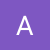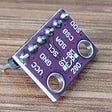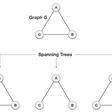# Obfuscating passwords using prime numbers and modular arithmetic

Some time ago I needed a complex password. However, I also wanted it to be something that I can remember. What’s easier to remember, “Password123456789” or “Uz8!;JNEw.B>vS?Q2”? So I decided to to put together my own obfuscater. Just for the fun of it.

My requirements were:

• Algorithm should run quickly
• Given one obfuscated password as input, a new and obfuscated password should be created. This way, I only need to remember one password, which can be used to generate many different, seemingly obscure passwords. Great for places requiring password changes often :)
• As extension of the previous requirement, the algorithm must generate many different passwords before returning a previously generated password

The rest of this post will give a little background on the Caesar cipher and how to use this as the basis for a more advanced obfuscater.

# Caesar cipher

This is a very old and well-known cipher. It is simply a substitution of letters by other letters n letters away. For instance, Caesar cipher with a shift of +4 would map “caezar” to “geidev”, because each letter is now replaced by the letter 4 letters later in the alphabet. In the case where we will go beyond the alphabet (what comes 4 letters after z in the alphabet?), we simply loop back to the start of the alphabet, so that z maps to d.
Decryption is the inverse operation. If we encoded with a shift of +4, then the decode with a shift of -4, so that “geidev” maps to “caezar”. Once more, if we get to the start of the alphabet, we continue from the end, so that “d” becomes “z”.
If we translate numbers into letters, such that a=>0, b=>1, …, z=>25, then we can write the encoding function E and decoding function D, for some shifting-amount of n:
E(x) = (x +n) mod 26
D(x) = (x -n) mod 26

An interesting special-case of the Caesar cipher is the case where n = 13. In this case, E(x) = D(x). Because there are 26 letters in the alphabet, if you rotate by 13 twice, you will end up with the starting word. You can read more about the Caesar cipher on Wikipedia.

# Improvement: Index-based Caesar cipher

In substitution-based ciphers (like Caesar cipher), one of the common attack-vectors is simply to perform a frequency analysis. This involves simply counting the different letters in the encrypted text and try to guess which letter is which. In English, the most common letter is “e”, so it makes sense that the most common letter in the encrypted text maps to “e”, and so on. Once more, you can read more on Wikipedia.

A different attack vector against Caesar cipher is when we know it’s encrypted with the Caesar cipher, but don’t know what the shifting-amount is. In this case, we can simply just try out different shifts and see what gives something that makes sense.

We can combat this by shifting the letters differently. E.g., the first letter is shifted by 4, second by 4*4 = 16, third by 4*4*4 = 64, and so on. This way, frequency analysis is no longer possible, since multiple letters may map to the same letter depending on their index. The second attack is still possible, given the attacker know about about the cipher. But since this isn’t a common cipher, it’s unlikely. This is an example of security by obscurity, but since the the goal is to build something that obfuscates passwords, and not an actual encryption scheme, this is fine :)

In general, the encryption and decryption functions are then:

E(x, i) = (x +n^i) mod 26
D(x, i) = (x -n^i) mod 26

Where indexes are 1-indexed. Working with modular exponents is known as modular exponentiation.

Let’s once more obfuscate the word “caezar” with 4 as the shifting-amount:
c: E(2, 1) = 2+4¹ mod 26 = 6
a: E(0, 2) = 0+4² mod 26 = 16
e: E(4, 3) = 4 + 4³ mod 26 = 16
z: E(25, 4) = 25 + 4⁴ mod 26 = 21
a: E(0, 5) = 0+4⁵ mod 26 = 10
r: E(17, 6) = 17+4⁶ mod 26 = 5
In all, this gives the obfuscated string “gqqvkf”. Interestingly, and as intended, “a” maps to two different values, and two different values map to the same value 16 (or the letter “q”).

# Shifting-amount cycles and alphabet size

Consider now the instance where we wish to obfuscate “caezarcaezar” by 4. We already know that the obfuscated string will start with “gqqvkf”. So let’s compute the remainder of the string:
c: E(2, 7) = 2 + 4⁷ mod 26 =6
a: E(0, 8) = 0 + 4⁸ mod 26 =16
e: E(4, 9) = 4 + 4⁹ mod 26 =16
z: E(25, 10) = 25 + 4¹⁰ mod 26 =21
a: E(0, 11) = 0 + 4¹¹ mod 26 =10
c: E(17, 12) = 17 + 4¹² mod 26 =5

Uh oh. It seems that we are beginning to repeat values. Calculating:
4^k mod 26 for different values of k yields why:
4¹ mod 26 = 4
4² mod 26 = 16
4³ mod 26 = 12
4⁴ mod 26 = 22
4⁵ mod 26 = 10
4⁶ mod 26 = 14
4⁷ mod 26 = 4
4⁸ mod 26 = 16
And so on. Indeed, we are caught in a cycle. This may not seem too bad, but it does add some predictability, which is what we didn’t want. However, we can’t avoid it completely; after-all, there can at most be 26 different values mod 26, after which it will repeat. So, we wish to choose a shifting-amount such that the cycle length is maximized. As it turns out, the longest possible cycle for the modulo 26 is of length 12 (which you get by using 7 for shift-value). This we know from group theory, as we, even though I haven’t talked about it, are actually working in what is known as a multiplicative group modulo n, with n being 26. Unless you’re interested in the math (if you are, go read the Wikipedia article), it’s mostly important to know that the longest cycle for a number n is equal to the number of integers less than n for which n shares no common divisors (this is known as Euler’s totient function). E.g., 26 = 2*13, so any even number less than 26 (there are 12) will share a divisor (2), 13 will share a divisor (13) and the trivial 1 will share a divisor, giving 14 numbers for which 26 shares a divisor. Indeed, 26–14 = 12, giving the longest possible cycle length.

To get longer cycles, we wish to work modulo a different number, preferably one such that there are no numbers below it for which it shares common divisors, except for the number 1, as this will minimize the number of numbers for which a common divisor is shared. It thus follows, that in order to optimize the cycle length, we must work modulo a prime number. And we can do that by extending our alphabet to also include the characters !/#, so that the size of the alphabet (lowercase letters and three special characters) is now of length 29, a prime number.
A note here is that not all shifting-amounts maximizes cycle lengths. Recall that 4 and 7 gave different lengths modulo 26. We can optimize by finding a number, n, such that modulo some prime number p:
n^((p-1)/2) mod p = p-1
If you wish to try, using the number 2 as the shifting-amount will give a cycle length of 28 for an alphabet of size 29. Verify this by calculating 2¹⁴ mod 29 = 28.

# Picking a number for shifting-amount

I touched upon how different shifting-amounts would yield different cycle lengths, and how we could simply pick another to get better cycle lengths. However, there is also a different and more threatening problem which is to be considered when choosing how much to shift by, and that is that we will quickly use the same numbers.
Consider once more the alphabet of lowercase English letters, in which there are 26 letters, and that we still use 4 for shifting. Let’s play around with the letter “caezar” once more and obfuscate them a few times:
- 0th iteration: caezar
- 1st iteration: gqqvkf

- 12th iteration: yksdqd
- 13th iteration: caezar
Aaand, once more, we get cycling. Again, we can’t avoid them (it is to be expected after 26 iterations), but by picking the number 4, I made a bad decision, namely picking a number that shared a divisor with 26 — the number 2. Had I instead picked 5, the cycling would happen only after 26 iterations, maximizing how many different strings we can generate from the original “caezar”.

So, once more, by ensuring that our alphabet is of a prime number size, we can pick pretty much freely for any shifting-amounts — except 1, because 1^n = 1, and so this will only give a simple Caesar cipher :)

# Putting it all together

Now with the background in place, we can put together an algorithm. But first, you need to choose which letters can be used, and make certain that the number of letters is a prime number. For example, you can use lowercase letters, uppercase letters and the number 0, giving 53 different letters to be used:
abcdefghijklmnopqrstuvwxyzABCDEFGHIJKLMNOPQRSTUVWXYZ0

Next, pick a number lower than the length of your allowed characters and greater than 1. Let’s pick the number 5, as it fits the criteria that:
5²⁶ mod 53 = 52

Now, in pseudo-code, we can obfuscate a password:
`function obfuscate(input: string, characters: char[])obfuscated_string = ""for i in range(0, input.length): — character = input[i] — nextCharacterIndex = characters.indexOf(character) + 5^(i+1) — nextCharacter = characters[nextCharacterIndex%characters.length]`
`— obfuscated_string = obfuscated_string+nextCharacterreturn obfuscated_string`

And that’s it! I’ve implemented the algorithm in JavaScript here, with 5 as the shifting-amount, and 83 allowed characters. Feel free to use the tool (at own risk, I won’t promise not to remove it in the future, so don’t depend on it), or just copy the source code and use it somewhere else.

# Optimizations

When working with modular arithmetic, we have the following rewriting rules:
a + b mod m = (a mod m) + (b mod m) mod m
a*b mod m = (a mod m) * (b mod m) mod m
This means that calculating 5⁴+3 mod 4 can be calculated as:
(5 mod 4)*(5 mod 4)*(5 mod 4)*(5 mod 4) + 3 mod 4
= 1*1*1*1 + 3 mod 4 = 0 mod 4
This removes the need for calculating 5⁴, and this really speeds up the calculation. This really comes into play if we were to calculate 23¹⁹, for instance, where that would give a very large number. One that would also overflow the integers, so good thing we can avoid that.

One more thing that I haven’t touched on, but just assumed up until now, is how the alphabet is sorted. Well, it’s sorted alphabetically, right? But what if instead of using abcdefghijklmnopqrstuvwxyz as alphabet, we were to use lobirvwkmjtczfsgpaenuxqhyd? Now, shifting the string “lo” using 3 for shifting-amount gives “it”, making the output look even more random.

# Conclusion

With the arithmetic rewrites from the optimizations section, the code runs very quickly. This satisfies the speed requirement. Obviously, encoding an obfuscated string gives a new, different string, satisfying my second requirement. Indeed, one can run an input through the obfuscater p times, for some prime number, before getting the original one — satisfying the last requirement. So now, I only need to remember my password “1337password” to have a lot, random-looking passwords generated. Awesome!

As a bonus, even though this was never the purpose, all the math used is reversible, meaning that we can also use it for encrypting and decrypting data. We shouldn’t actually do it, and making and using your own encryption algorithm is a bad idea, but for the case of obfuscating passwords using a custom obfuscater that no-one else knows about, the algorithm is sound enough.

This concludes the background and implementation of my custom obfuscater. The whole point of it was to try to apply some knowledge on abstract algebra with a real world usage. You might wonder: why not just use a simpler method, like just using a common hashing algorithm like MD5 or SHA256? Well, that wouldn’t have been as much fun as this :)

--

--

--

## More from Andreas Thiele

Love podcasts or audiobooks? Learn on the go with our new app.

## Why hackers are targeting the supply chain## TryHackMe: Blue## Understanding online fraud## Is Netflix Changing Our Perception of Cybersecurity?## PSA Certified## CodeChef RTU Chapter## Project 4: ESP 32’s External Sensor## How to Make a Interactive Camera using Cinemachine and C### Prim’s Algorithm